## Available courses

### SY VAC AI in Healthcare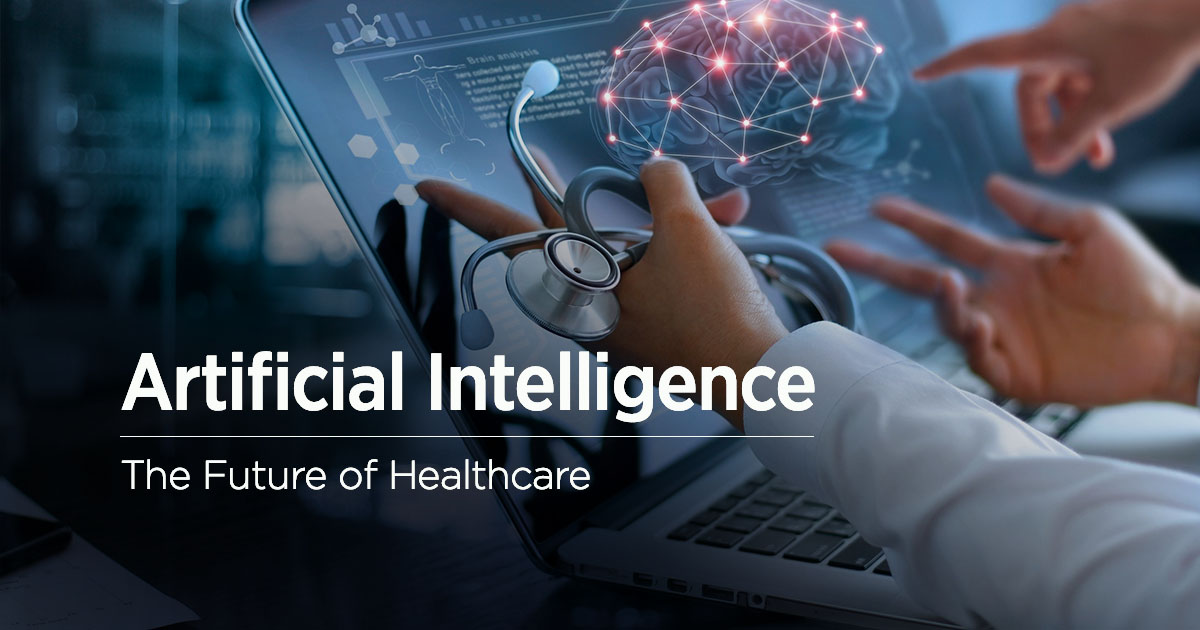Certificate Course in Artificial Intelligence for Healthcare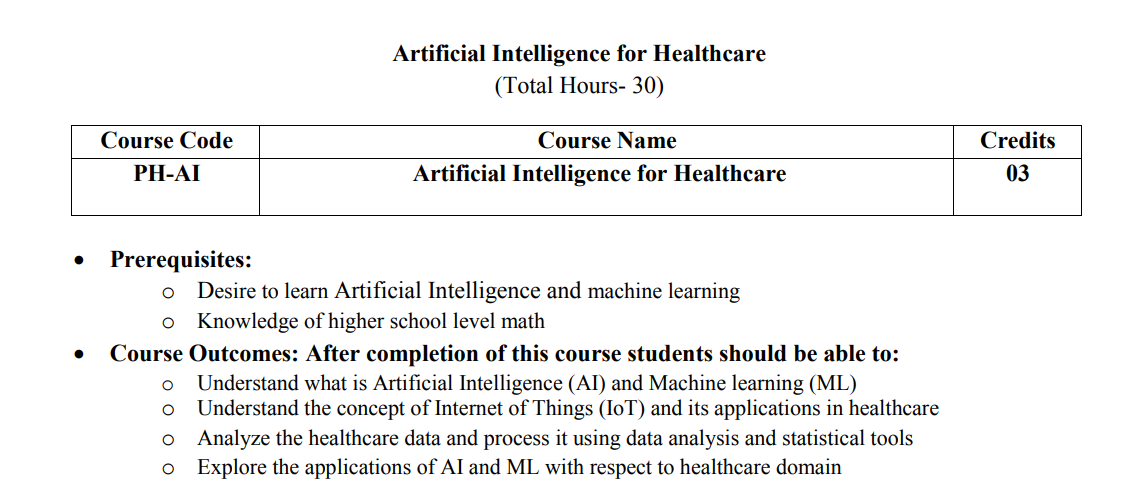### Data Science Using Python Submission

Data Science Using Python Submission course is a course for performing submission of ITL604 Lab for AY 2022-23 Batch of IT Department.

### EM_IV_IT_2223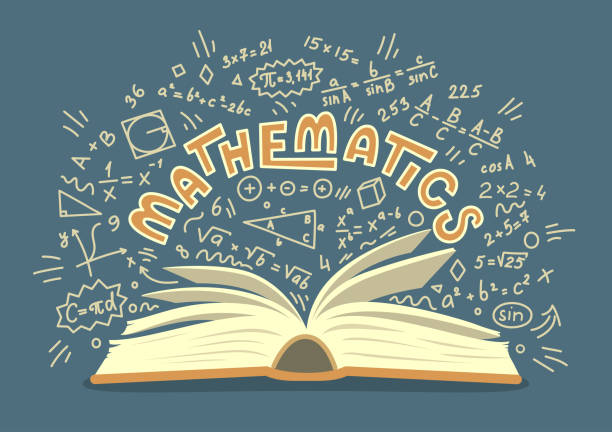### Automata Theory

Automata Theory involves study of Abstract Mathematical representation of computational devices having varied organization and formally prove their capability of solving certain categories of computational problems.

We start with learning about the abstraction called as Language (Formal Language) Which is used by researchers to categorize computational problems into 4 categories Regular, Context Free, Context Sensitive, Recursively Enumerable. We also dedicate our attention to mathematical devices called as FA, PDA, TM.

### Mini Project – 1 A for Front end /backend Application using JAVA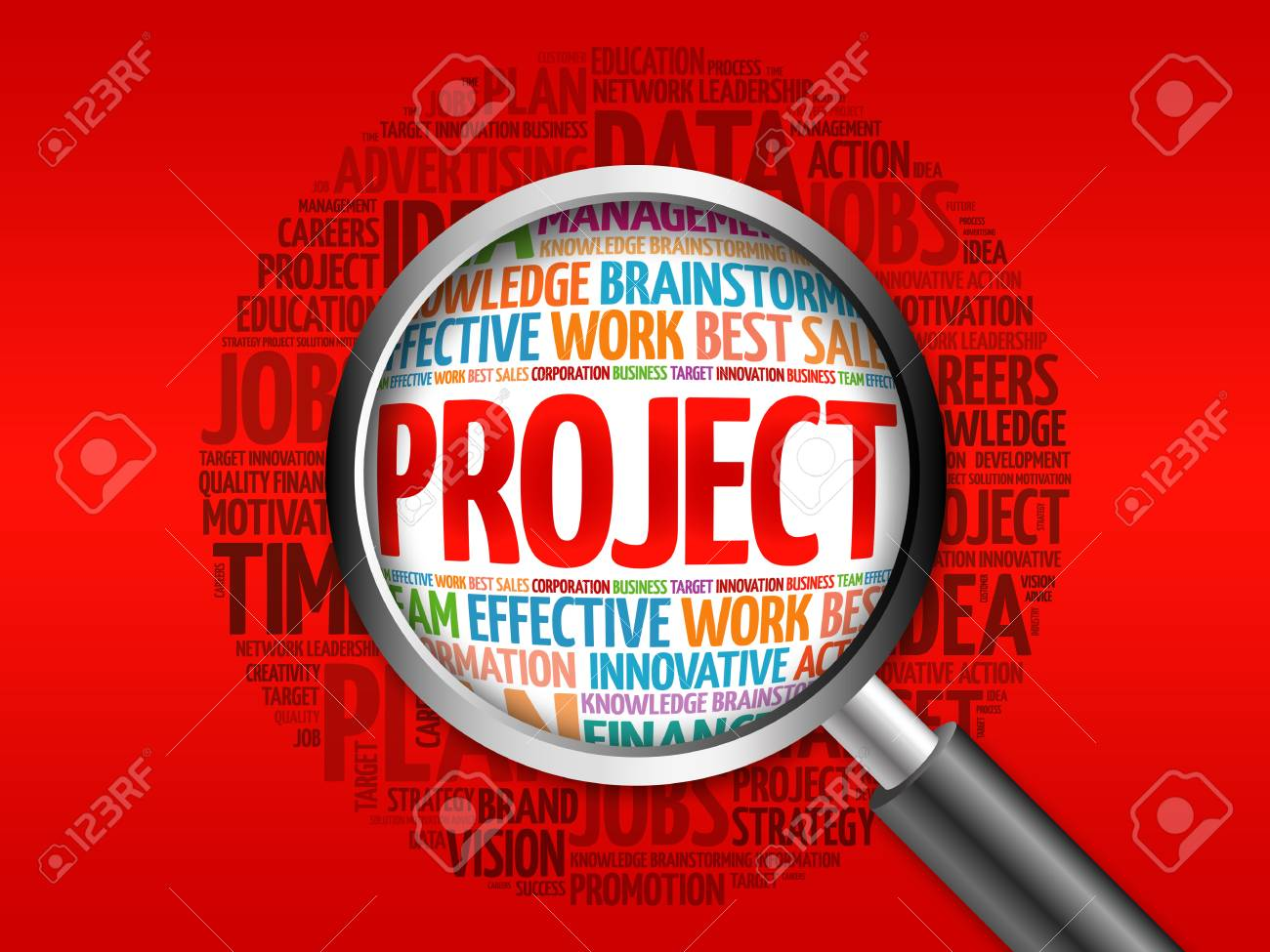Mini Project – 1 A for Front end/back end Application using JAVA

Course Objectives
1. To acquaint with the process of identifying the needs and converting it into the problem.
2. To familiarize the process of solving the problem in a group.
3. To acquaint with the process of applying basic engineering fundamentals to attempt solutions to the problems.
4. To inculcate the process of self-learning and research.
Course Outcome:
Learner will be able to...
1. Identify problems based on societal /research needs.
2. Apply Knowledge and skill to solve societal problems in a group.
3. Develop interpersonal skills to work as member of a group or leader.
4. Draw the proper inferences from available results through theoretical/ experimental/simulations.
5. Analyze the impact of solutions in societal and environmental context for sustainable development.
6. Use standard norms of engineering practices
7. Excel in written and oral communication.
8. Demonstrate capabilities of self-learning in a group, which leads to life long learning.
9. Demonstrate project management principles during project work.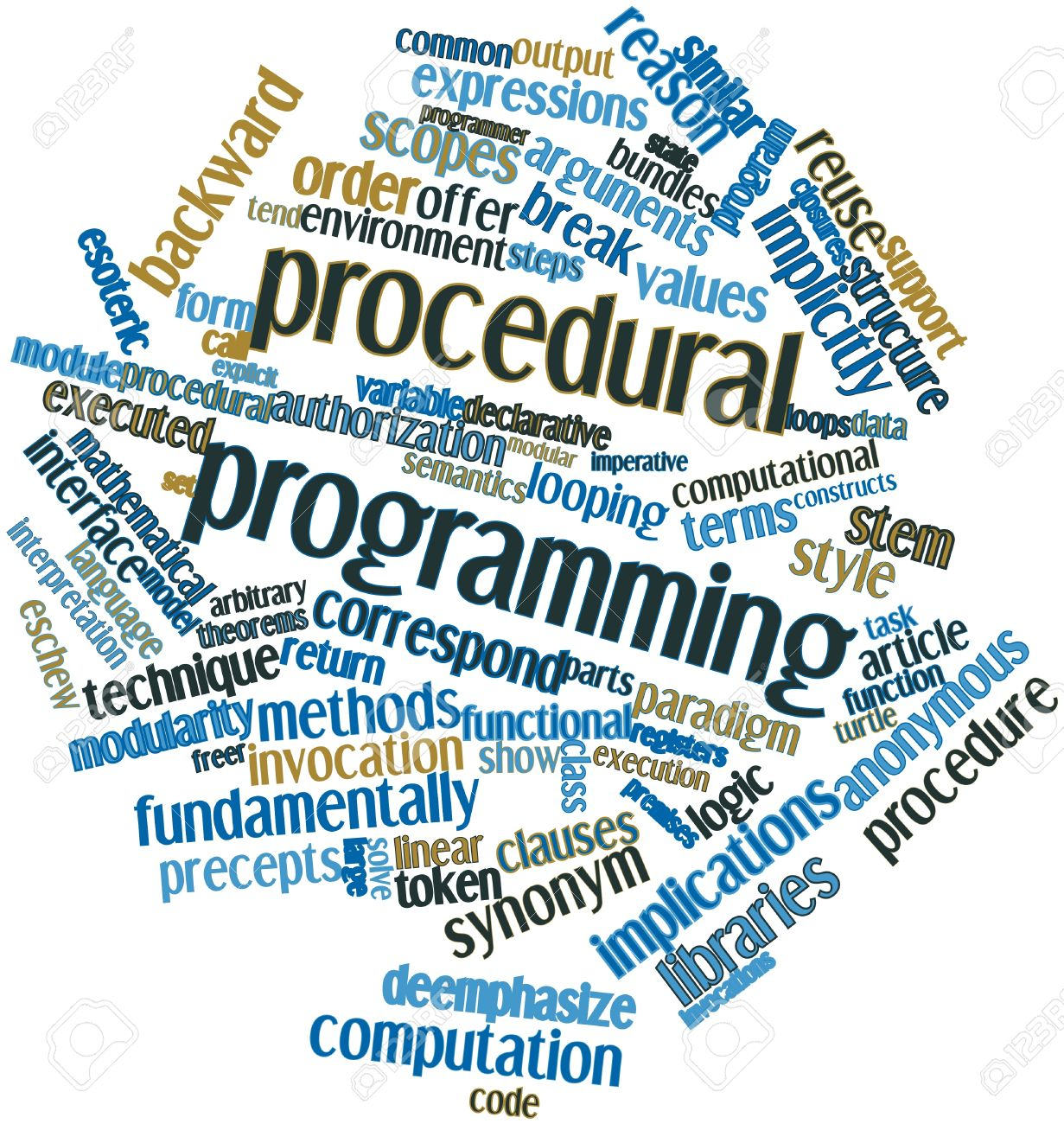Lab Objectives:

The Lab experiments aims:
1. Understand data abstraction and object orientation
2. Design and implement declarative programs in functional and logic programming languages
3. Introduce the concepts of concurrent program execution
4. Understand run time program management
5. Understand how to implement a programming solution using different programming paradigms.
6. Learn to compare implementation in different programming paradigms.
Lab Outcomes:

On successful completion, of course, learner/student will be able to:
1. Implement Object Oriented concepts in C++. L1, L2, L3
2. Design and Develop solution based on declarative programming paradigm using functional and logic programming. L6
3. Understand the multi threaded programs in Java and C++ L1, L2
4. Understand the need and use of exception handling and garbage collection in C++ and JAVA L2, L3
5. Implement a solution to the same problem using multiple paradigms. L6
6. Compare the implementations in multiple paradigms at coding and execution level. L4

### SQL Lab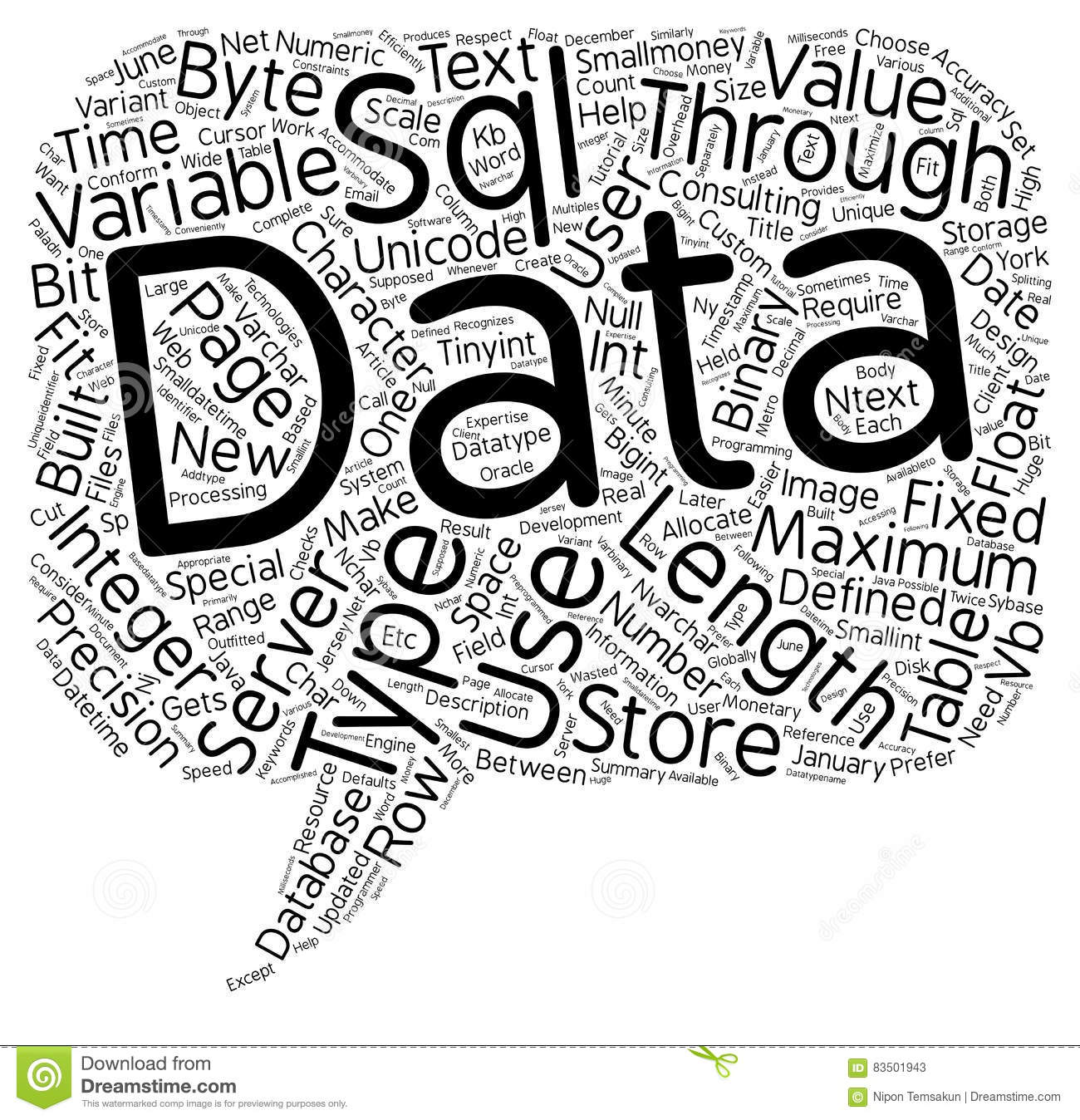SQL Lab

Lab Objectives:

The Lab experiments aims:

1. To identify and define problem statements for real life applications
2. To construct conceptual data model for real life applications
3. To Build Relational Model from ER/EER and demonstrate usage of relational algebra.
4. To Apply SQL to store and retrieve data efficiently
5. To implement database connectivity using JDBC
6. To understand the concepts of transaction processing- concurrency control & recovery procedures.
Lab Outcomes:
On successful completion, of course, learner/student will be able to:
1. Define problem statement and Construct the conceptual model for real life application. L1, L3, L4, L6
2. Create and populate a RDBMS using SQL. L3, L4
3. Formulate and write SQL queries for efficient information retrieval L3, L4
4. Apply view, triggers and procedures to demonstrate specific event handling. L1, L3, L4
5. Demonstrate database connectivity using JDBC. L3
6. Demonstrate the concept of concurrent transactions. L3, L4

### Data Structure Lab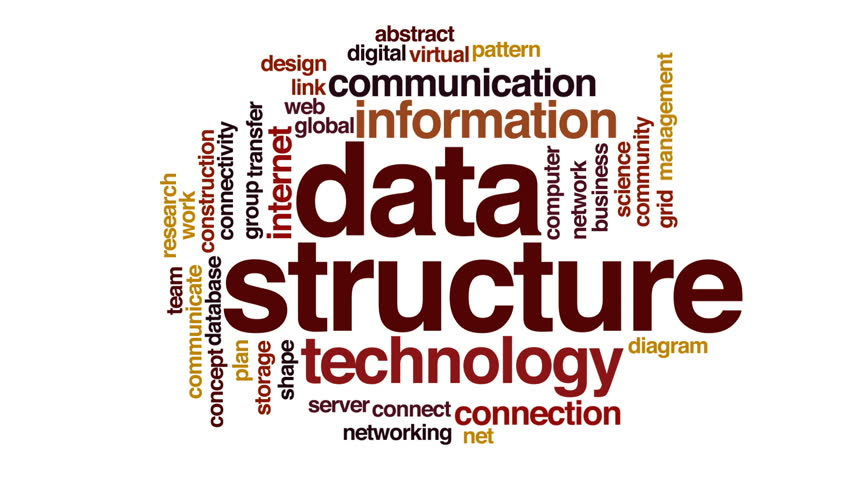Data Structures Lab

Lab Objectives:

The Lab experiments aims:

1. To use data structures as the introductory foundation for computer automation to engineering problems.
2.  To use the basic principles of programming as applied to complex data structures.
3. To learn the principles of stack, queue, linked lists and its various operations.
4. To learn fundamentals of binary search tree, implementation and use of advanced tree like AVL, B trees and graphs.
5. To learn about searching, hashing and sorting.
6. To learn the applications of linked lists, stacks, queues, trees and graphs.
Lab Outcomes:

On successful completion, of course, learner/student will be able to:
1. Understand and use the basic concepts and principles of various linked lists, stacks and queues. L1, L2, L3
2. Understand the concepts and apply the methods in basic trees. L1, L2
3. Use and identify the methods in advanced trees. L3, L4
4. Understand the concepts and apply the methods in graphs. L2, L3
5. Understand the concepts and apply the techniques of searching, hashing and sorting L2, L3
6. Illustrate and examine the methods of linked lists, stacks, queues, trees and graphs to various real time problems L3, L4

### Paradigms and Computer Programming Fundamentals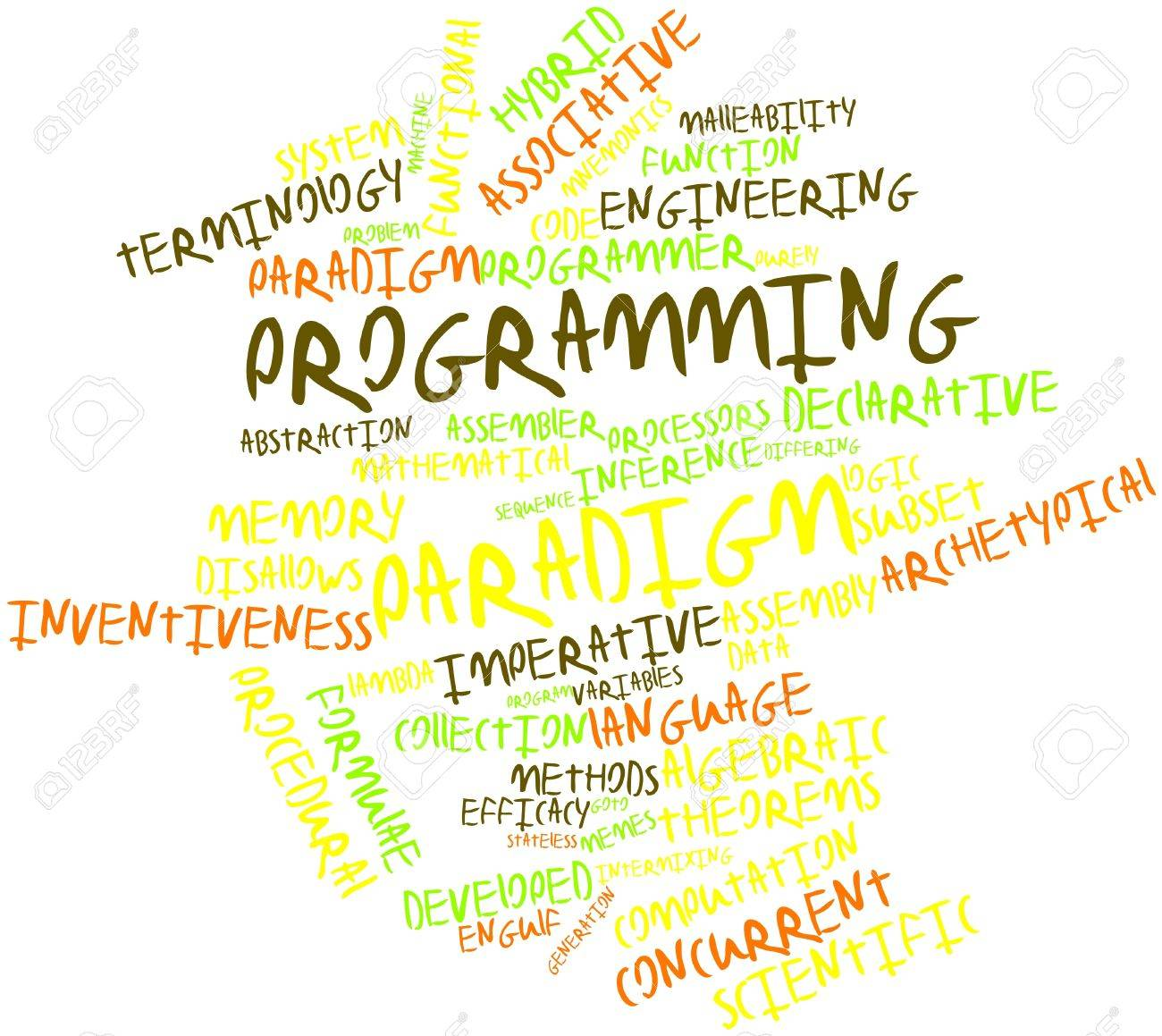Course Objectives:

The course aims:

1. To introduce various programming paradigms and the basic constructs that underline any  programming language.
2. To understand data abstraction and object orientation
3. To introduce the basic concepts of declarative programming paradigms through functional and logic programming.
4. To design solutions using declarative programming paradigms through functional and logic programming
5. To introduce the concepts of concurrent program execution.
6. To understand use of scripting language for different problem domains

### Principle of CommunicationPrinciple of Communication

Course Objectives:

The course aims:

1. Study the basic of Analog and Digital Communication Systems.
2. Describe the concept of Noise and Fourier Transform for analyzing communication systems.
3. Acquire the knowledge of different modulation techniques such as AM, FM and study the block diagram of transmitter and receiver.
4. Study the Sampling theorem and Pulse Analog and digital modulation techniques
5. Learn the concept of multiplexing and digital band pass modulation techniques
6. Gain the core idea of electromagnetic radiation and propagation of waves.
Course Outcomes:
On successful completion, of course, learner/student will be able to:
1. Describe analog and digital communication systems L1,L2
2. Differentiate types of noise, analyses the Fourier transform of time and frequency domain. L1, L2, L3, L4
3. Design transmitter and receiver of AM, DSB, SSB and FM. L1,L2,L3,L4
4. Describe Sampling theorem and pulse modulation systems. L1,L2,L3
5. Explain multiplexing and digital band pass modulation techniques. L1, L2
6. Describe electromagnetic radiation and propagation of waves. L1,L2

### Database Management System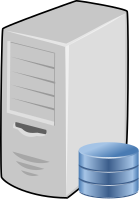Database Management System

Course Objectives:

The course aims:

1.  To learn the basics and understand the need of database management system.
2. To construct conceptual data model for real world applications
3. To Build Relational Model from ER/EER.
4. To introduce the concept of SQL to store and retrieve data efficiently.
5. To demonstrate notions of normalization for database design.
6. To understand the concepts of transaction processing- concurrency control & recovery procedures.
Course Outcomes:

On successful completion, of course, learner/student will be able to:

1. Identify the need of Database Management System. L1, L2
2. Design conceptual model for real life applications. L6
3. Create Relational Model for real life applications L6
4. Formulate query using SQL commands. L3
5. Apply the concept of normalization to relational database design. L3
6. Demonstrate the concept of transaction, concurrency and recovery. L2

### Data Structure and AnalysisCourse Objectives:

The course aims:

1. The fundamental knowledge of data structures.
2. The programming knowledge which can be applied to sophisticated data structures.
3. The fundamental knowledge of stacks queue, linked list etc.
4. The fundamental knowledge of Trees, Graphs etc
5. The fundamental knowledge of different sorting, searching, hashing and recursion techniques
6. The real time applications for stacks, queue, linked list, trees, graphs etc
Course Outcomes:

On successful completion, of course, learner/student will be able to:

1. 1 Classify and Apply the concepts of stacks, queues and linked list in real life problem solving.L1, L2, L3
2. Classify, apply and analyze the concepts trees in real life problem solving. L2, L3,L4
3. Illustrate and justify the concepts of graphs in real life problem solving. L3, L5
4. List and examine the concepts of sorting, searching techniques in real life problem solving. L2, L3, L4
5. Use and identify the concepts of recursion, hashing in real life problem solving. L3, L4
6. Examine and justify different methods of stacks, queues, linked list, trees and graphs to various applications. L3, L4, L5

### Engineering Mathematics -III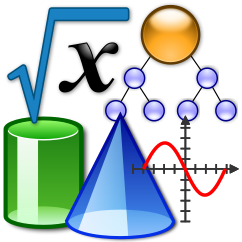Course Objectives:

1. To familiarize with the Laplace Transform, Inverse Laplace Transform of various functions, and its applications.
2.  To acquaint with the concept of Fourier series, its complex form and enhance the problem solving skills.
3. To familiarize the concept of complex variables, C-R equations with applications.
4. The fundamental knowledge of Trees, Graphs etc.
5. To study the basic techniques of statistics like correlation, regression and curve fitting for data analysis, Machine learning and AI.
6. To understand some advanced topics of probability, random variables with their distributions and expectations.
Course Outcomes:
On successful completion, of course, learner/student will be able to:
1. Apply the concept of Laplace transform to solve the real integrals in engineering problems.   (Blooms Level L1, L2)
2. Apply the concept of inverse Laplace transform of various functions in engineering problems. (Blooms Level L1, L2)
3. Expand the periodic function by using Fourier series for real life problems and complex engineering problems. (Blooms Level L1, L2, L3)
4. Find orthogonal trajectories and analytic function by using basic concepts of complex variable theory. (Blooms Level L1, L2, L3)
5. Apply the concept of Correlation and Regression to the engineering problems in data science, machine learning and AI. (Blooms Level: L2, L3)
6. Illustrate understanding of the concepts of probability and expectation for getting the spread of the data and distribution of probabilities. (Blooms Level L1, L2)

### Linux Command Line and Bash scripting Workshop 1

Workshop companion resources for Linux Command Line and Bash Script Basics.

### ENGG.MATHS-III COMP 2022-23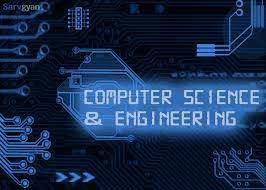### Engineering Mathematics-III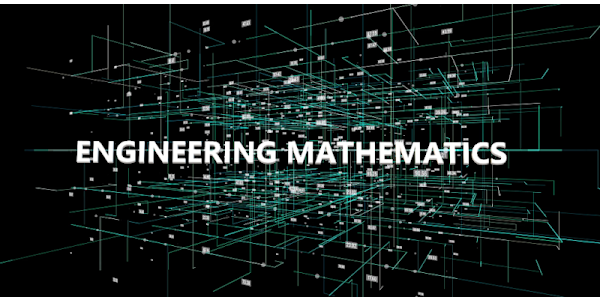Course Objectives: The course aims:
1. To learn the Laplace Transform, Inverse Laplace Transform of various functions, its applications.
2. To understand the concept of Fourier Series, its complex form and enhance the problem-solving skills.
3. To understand the basic techniques of statistics like correlation, regression, and curve fitting for data analysis, Machine learning, and AI.
4. To understand some advanced topics of probability, random variables with their distributions and expectations

Course Outcomes: On successful completion, of course, learner/student will be able to:

1. Understand the concept of Laplace transform and its application to solve the real integrals in engineering problems.
2. Understand the concept of inverse Laplace transform of various functions and its applications in engineering problems.
3. Expand the periodic function by using the Fourier series for real-life problems and complex engineering problems.
4. Understand complex variable theory, application of harmonic conjugate to get orthogonal trajectories and analytic functions.
5. Apply the concept of Correlation and Regression to the engineering problems in data science, machine learning, and AI.
6. Understand the concepts of probability and expectation for getting the spread of the data and distribution of probabilities.

### ENGG.MATHS-III MECH 2022-23Objectives: The course is aimed

1. To familiarize with the Laplace Transform, Inverse Laplace Transform of various functions, its applications.

2. To acquaint with the concept of Fourier Series, its complex form and enhance the problem solving skills

3. To familiarize with the concept of complex variables, C-R equations with applications.

4. To study the application of the knowledge of matrices and numerical methods in complex engineering problems.

Outcomes: On successful completion of course learner/student will be able to:

1. Apply the concept of Laplace transform to solve the real integrals in engineering problems.

2. Apply the concept of inverse Laplace transform of various functions in engineering problems.

3. Expand the periodic function by using Fourier series for real life problems and complex engineering problems.

4. Find orthogonal trajectories and analytic function by using basic concepts of complex variable theory.

5. Apply Matrix algebra to solve the engineering problems.

6. Solve Partial differential equations by applying numerical solution and analytical methods for one dimensional heat and wave equations.

### Engineering Mathematics-III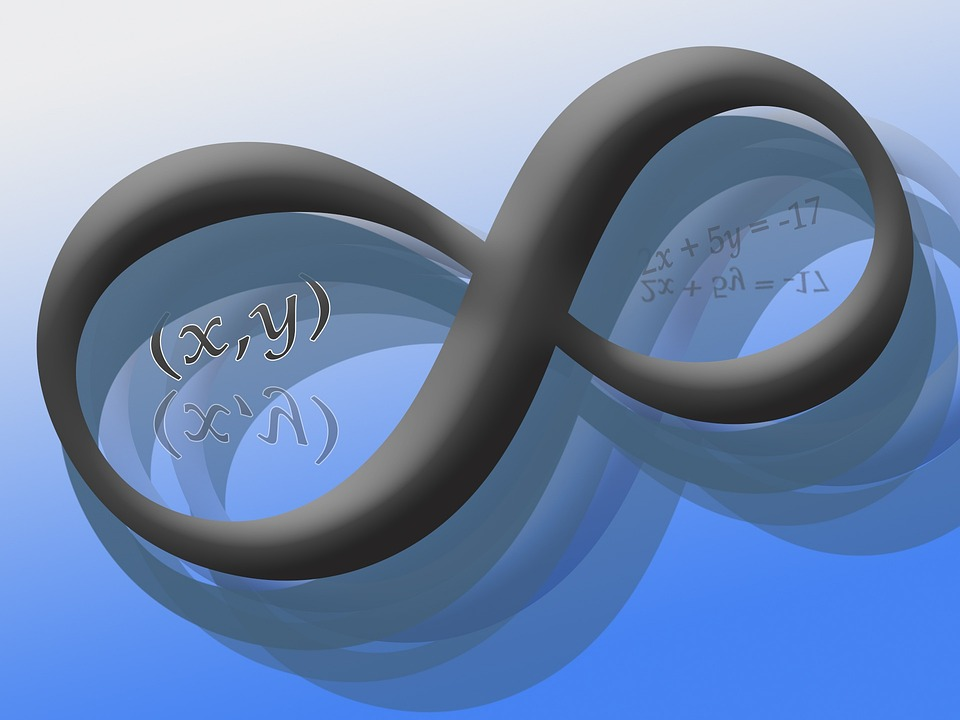Course Objectives: The course aims:
1. To learn the Laplace Transform, Inverse Laplace Transform of various functions, its applications.
2. To understand the concept of Fourier Series, its complex form and enhance the problem-solving skills.
3. To understand the basic techniques of statistics like correlation, regression, and curve fitting for data analysis, Machine learning, and AI.
4. To understand some advanced topics of probability, random variables with their distributions and expectations

Course Outcomes: On successful completion, of course, learner/student will be able to:

1. Understand the concept of Laplace transform and its application to solve the real integrals in engineering problems.
2. Understand the concept of inverse Laplace transform of various functions and its applications in engineering problems.
3. Expand the periodic function by using the Fourier series for real-life problems and complex engineering problems.
4. Understand complex variable theory, application of harmonic conjugate to get orthogonal trajectories and analytic functions.
5. Apply the concept of Correlation and Regression to the engineering problems in data science, machine learning, and AI.
6. Understand the concepts of probability and expectation for getting the spread of the data and distribution of probabilities.

### LMS Training WorkshopIt was decided to adopt Moodle 4.1 LMS as part of  teaching learning process during AY 22-23 ODD semester for second year semester 3 classes, all branches. A workshop in online mode was conducted on 23rd July 2022, by Prof. A S Kunte 02:30 PM to 04:00 PM.

The objective of workshop was to cover following points:

1. Explain role of Moodle LMS as a productivity improvement tool for Teaching Learning Process.
2. Make faculty members aware of login credentials for https://www.kgcelms.in
3.  Introduce them to various login levels for the site : Student, Teacher, Non Editing Teacher, Manager and Administrator.
4. Demonstrate course creation steps.
5. Introduce Assignment, Quiz and Page activity tools.

This

### Recent Open Source projects Lab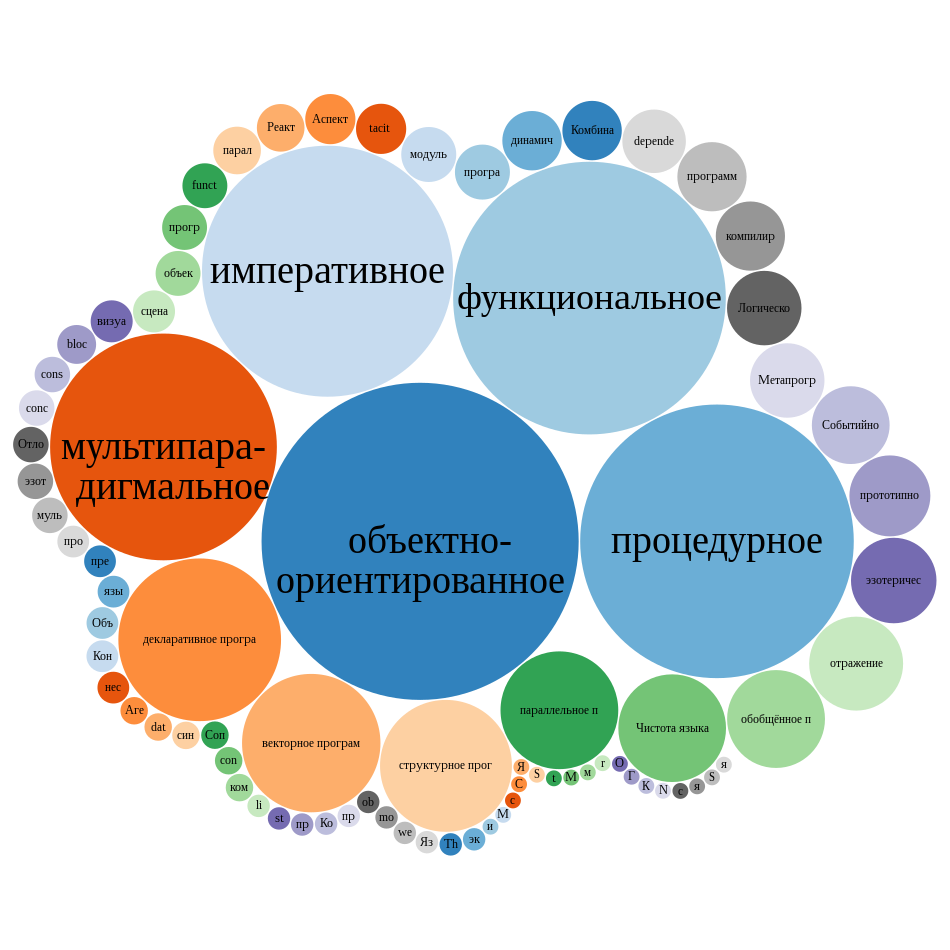This is Course Content for CPPL (Computer Programming Paradigms Lab) course from SEIt SEM III

### Data Science Lab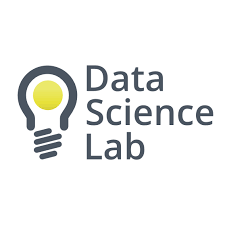The course has Six modules namely:

1. Uncertainty in AI ( 1 Practical)
2. Cognitive Computing (1 Practical)
3. Fuzzy Logic & Its Applications (1 Practical)
4. Introduction to Deep Learning (3 Practical)
5. Advanced ML Classification Techniques (2 Practicals)
6. Mini-project on trends and applications in Data Science (Mini Project Report)

The Lab involves  Completion of above said practical sessions During ODD SEM. We have organised this course to contain 6 Modules that need to completed each week by all batches and write ups need to be submitted in written or typed format as asked in assignments in every Module.

### Dev Ops and Adv Dev Ops Lab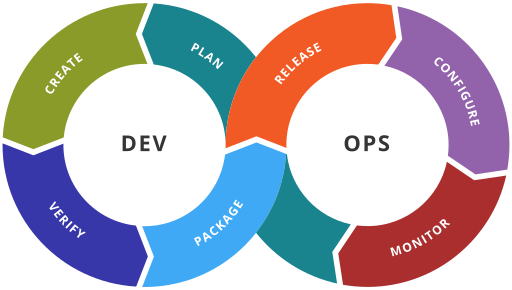During these courses we :

• To understand DevOps practices which aims to simplify Software Development Life Cycle
• To be aware of different Version Control tools like GIT, CVS or Mercurial
• To Integrate and deploy tools like Jenkins and Maven, which is used to build, test and deploy applications in DevOps environment
• To be familiarized with selenium tool, which is used for continuous testing of applications deployed.
• To use Docker to Build, ship and manage applications using containerization
• To understand the concept of Infrastructure as a code and install and configure Ansible tool.
• To understand DevOps practices and cloud native environments to achieve continuous software delivery pipelines and automated operations that address the gap between IT resources and growing cloud complexity.
• To Use Kubernetes services to structure N-tier applications.
• To be familiarized with Infrastructure as code for provisioning, compliance, and management of any cloud infrastructure, and service.
• To understand that security and speed in software development are not inversely-related objectives Internalizing the contribution of tools and automation in DevSecOps
• To understand various troubleshooting techniques by monitoring your entire infrastructure and business processes
• To understand how software and software-defined hardware are provisioned dynamically.

### Java Lab (SBL)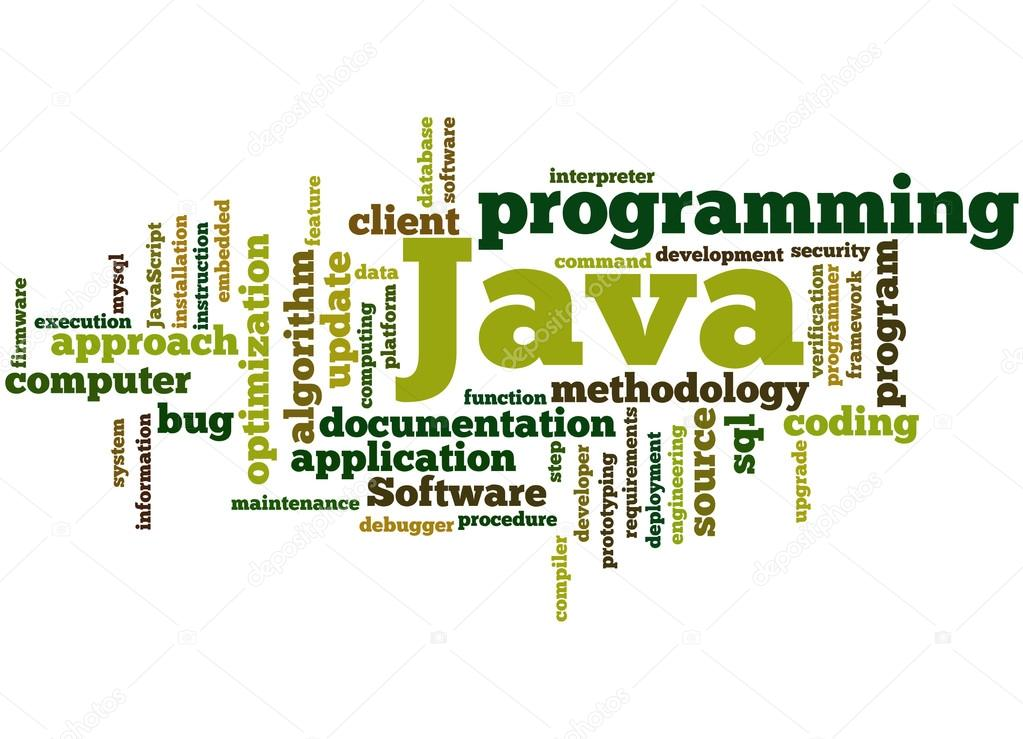Java Lab (SBL)

Lab Objectives:

The Lab experiments aims:

1. To understand the concepts of object-oriented paradigm in the Java programming language.
2. To understand the importance of Classes & objects along with constructors, Arrays ,Strings and vectors
3. To learn the principles of inheritance, interface and packages and demonstrate the concept of re-usability for faster development.
4. To recognize usage of Exception Handling, Multi threading, Input Output streams in various applications
5. To learn designing, implementing, testing, and debugging graphical user interfaces in Java using Swings and AWT components that can react to different user events.
6. To develop graphical user interfaces using JavaFX controls.
Lab Outcomes:

On successful completion, of course, learner/student will be able to:
1. Explain the fundamental concepts of Java Programing. L1, L2
2. Use the concepts of classes, objects, members of a class and the relationships among them needed for a finding the solution to specific problem. L3
3. Demonstrate how to extend java classes and achieve re-usability using Inheritance, Interface and Packages. L3
4. Construct robust and faster programmed solutions to problems using concept of Multi threading, exceptions and file handling L3
5. Design and develop Graphical User Interface using Abstract Window Toolkit and Swings along with response to the events. L6
6. Develop Graphical User Interface by exploring JavaFX framework based on MVC architecture. L6